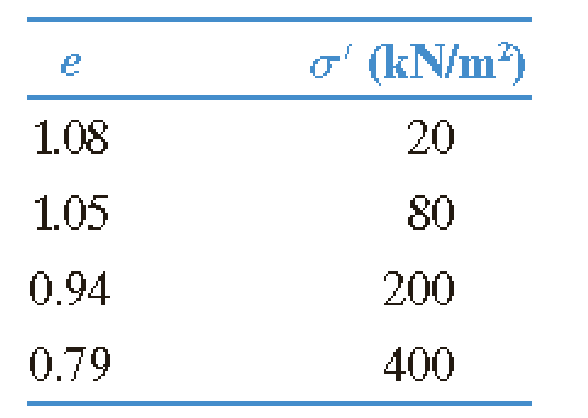Chapter 11, Problem 11.13PPrinciples of Geotechnical Enginee...

9th Edition
Braja M. Das + 1 other
ISBN: 9781305970939

Solutions

Chapter
SectionPrinciples of Geotechnical Enginee...

9th Edition
Braja M. Das + 1 other
ISBN: 9781305970939
Textbook Problem

A 4-in clay layer in the field has a current effective stress of σ ′ 0 = 58   kN / m 2 . There is a net stress increase of Δ σ = 195   kN / m 2 due to a foundation load. Only four data points are available from a consolidation test on the clay, as shown. Estimate the primary consolidation settlement of the clay layer in the field.To determine

Calculate the primary consolidation settlement of the clay layer.

Explanation

Given information:

The thickness of the clay layer (H) is 4m.

The effective stress (σ0) is 58kN/m2.

The net stress increase (Δσ) is 195kN/m2.

The results (pressure and void ratio) of the data points are given in the Table.

Calculation:

Sketch the elogσ graph using the Table values.

Show the elogσ curve as in Figure 1.

Refer to Figure 1.

Calculate the void ratio (e1) as shown below.

Take the value of e1 as 1.06, for the value σ0 of 58kN/m2.

Calculate σ0+Δσ as shown below.

Substitute 58kN/m2 for σ0 and 195kN/m2 for Δσ.

σ0+Δσ=58+195=253kN/m2

Calculate the void ratio (e2) as shown below

Still sussing out bartleby?

Check out a sample textbook solution.

See a sample solution

The Solution to Your Study Problems

Bartleby provides explanations to thousands of textbook problems written by our experts, many with advanced degrees!

Get Started

What are the main components of a database system?

Database Systems: Design, Implementation, & Management

Describe the operation of a power assisted recirculating ball gearbox.

Automotive Technology: A Systems Approach (MindTap Course List)

What is the purpose of decision tables? How do you create them?

Systems Analysis and Design (Shelly Cashman Series) (MindTap Course List)

Describe the differences between pipe and tubing.

Welding: Principles and Applications (MindTap Course List)

Explain whether or not a mobile device is a computer.

Enhanced Discovering Computers 2017 (Shelly Cashman Series) (MindTap Course List)